GeeksforGeeks App
Open AppBrowser
Continue

## Related Articles

Vector is a module in Rust that provides the container space to store values. It is a contiguous resizable array type, with heap-allocated contents. It is denoted by Vec<T>. Vectors in Rust have O(1) indexing and push and pop operations in vector also take  O(1) complexity. Vectors ensure they never allocate more than isize::MAX bytes.

A simple way of explaining a vector is that it is a container that stores the values like an array, but it has more advantages than an array data structure. It can be increase size dynamically during runtime. It provided by the standard library that can be store the value of any data type. The data of the vector is allocated on the heap. Its length defines the number of elements present in the vector. Its capacity defines the actual allocated space on the heap of this vector that is in the form of 2^n.

Syntax:

```Vec<T>

Where T denotes type of data.```

## Creating a vector in Rust:

To create Vector simply follow the below-listed methods.

### 1. Using Vec::new() Method:

`let v : Vec<i64> = Vec::new();  `

Here v is the initialized vector that will contain the 64-bit integer datatype. It is initialized with help of the Vec::new() method.

## Rust

 `fn` `main() {` `    ``let` `v : Vec = Vec::new(); ` `    ``// printing the size of vector``    ``println!(``"{ }"``,v.len());``}`

Output: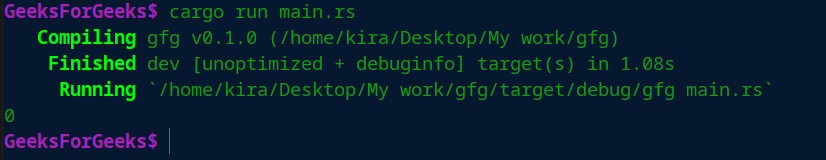### 2. Using macro in Rust:

`let v = vec!['G','E','E','K','S'];   `

Here this vector created using the macro vec!.  And it stores the value that we provide here which is char type.

## Rust

 `fn` `main() {` `    ``let` `v = vec![``'G'``,``'E'``,``'E'``,``'K'``,``'S'``];` `    ``// printing the size of vector``    ``println!(``"{ }"``,v.len());``}`

Output: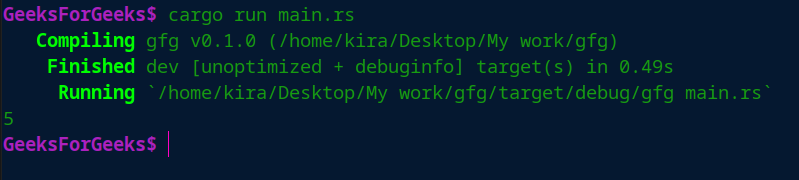## Accessing a Vector:

The below-listed methods can be used to access a vector in Rust programming.

### 1. Using subscript operator:

Similar to the concept of indexing in other languages, subscript operators can be used to directly access the values in a vector through their index. it is important to note that the indexing starts with 0.

```let v = vec!['G','E','E','K;','S'];

//here index is the integer non negative value which is smaller than the size of the vector.
char ch = v [ index ];```

Example:

## Rust

 `fn` `main() {` `    ``let` `v = vec![``'G'``,``'E'``,``'E'``,``'K'``,``'S'``];` `    ``// here index is the non negative value which is smaller than the size of the vector``    ``let` `index: usize = 3;` `    ``//getting value at given index value``    ``let` `ch: ``char` `= v[index];` `    ``print!(``"{ }\n"``,ch);``}`

Output: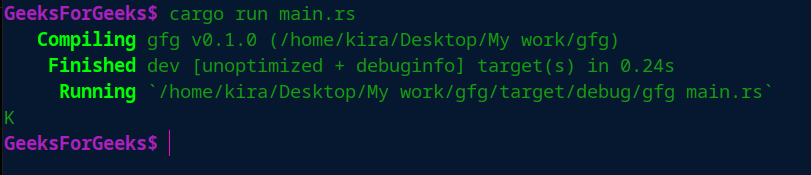### 2. Using the get() method

The second way of accessing the vector elements is to use the get(index) method with the index of a vector is passed as an argument. It returns the value of type Option<&t>.

```let v = vec!['G','E','E','K;','S'];

//here index is the integer non negative value which is smaller than the size of the vector.
let value: Option<&char> = v.get(index);  ```

Example:

## Rust

 `// Method to print the get value``fn` `value(n:Option<&``char``>) ``{ ``    ``match` `n ``    ``{ ``        ``Some(n)=>println!(``"Element of vector {}"``,n), ``        ``None=>println!(``"None"``), ``    ``} ``}` `fn` `main() {` `    ``let` `v = vec![``'G'``,``'E'``,``'E'``,``'K'``,``'S'``];` `    ``// here index is the non negative value which is``    ``// smaller than the size of the vector``    ``let` `index: usize = 3;` `    ``// getting value at given index value``    ``let` `ch: Option<&``char``> = v.get(index);``    ``value(ch);``}`

Output: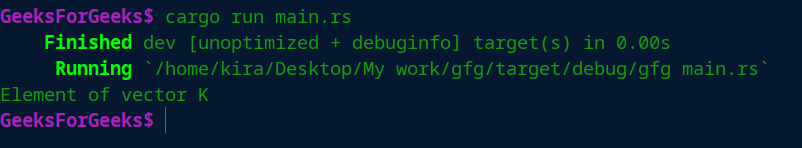### 3. Iterating on the vector:

To access the vector we can also iterate through a vector-like we do in other programming languages. We can use for loop to iterate through a vector.

```let v = vec!['G','E','E','K;','S'];

print!("Vector elements :");

for i in v
{
// iterating through i on the vector
print!("{} ",i);
}  ```

Example:

## Rust

 `fn` `main() {` `    ``let` `v = vec![``'G'``,``'E'``,``'E'``,``'K'``,``'S'``];` `    ``print!(``"Vector elements : "``); ` `    ``//loop to iterate elements in vector``    ``for` `i ``in` `v ``    ``{ ``        ``// iterating through i on the vector``        ``print!(``"{} "``,i); ``    ``} ``    ``print!(``"\n"``,);``}`

Output: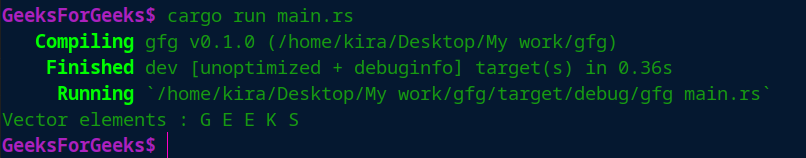## Updating a Vector:

After the creation of the vector, we can update the vector using the push() method. It pushes the new element if vector size is the less than the vector capacity or if vector size is more than vector capacity then it assigns the space double of vector size, then it copies all the elements in the newly assigned vector and releases the memory of the previous vector.

It pushes the element at the end of the vector.

```let mut v : Vec<char> = Vec::new();

vec.push('A');
vec.push('B');
vec.push('C');
// now vector size will be 3 ```

Example:

## Rust

 `fn` `main() {` `    ``let` `mut` `v : Vec<``char``> = Vec::new();` `    ``v.push(``'A'``);``    ``v.push(``'B'``);``    ``v.push(``'C'``);``    ``// now vector elements will be` `    ``// loop to iterate elements in vector``    ``for` `i ``in` `v ``    ``{ ``        ``// iterating through i on the vector``        ``print!(``"{} "``,i); ``    ``} ``    ``print!(``"\n"``);``}`

Output: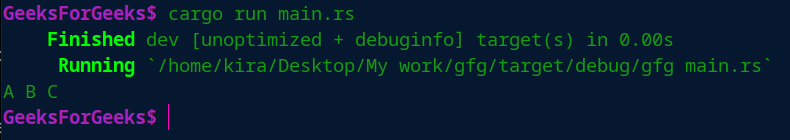My Personal Notes arrow_drop_up